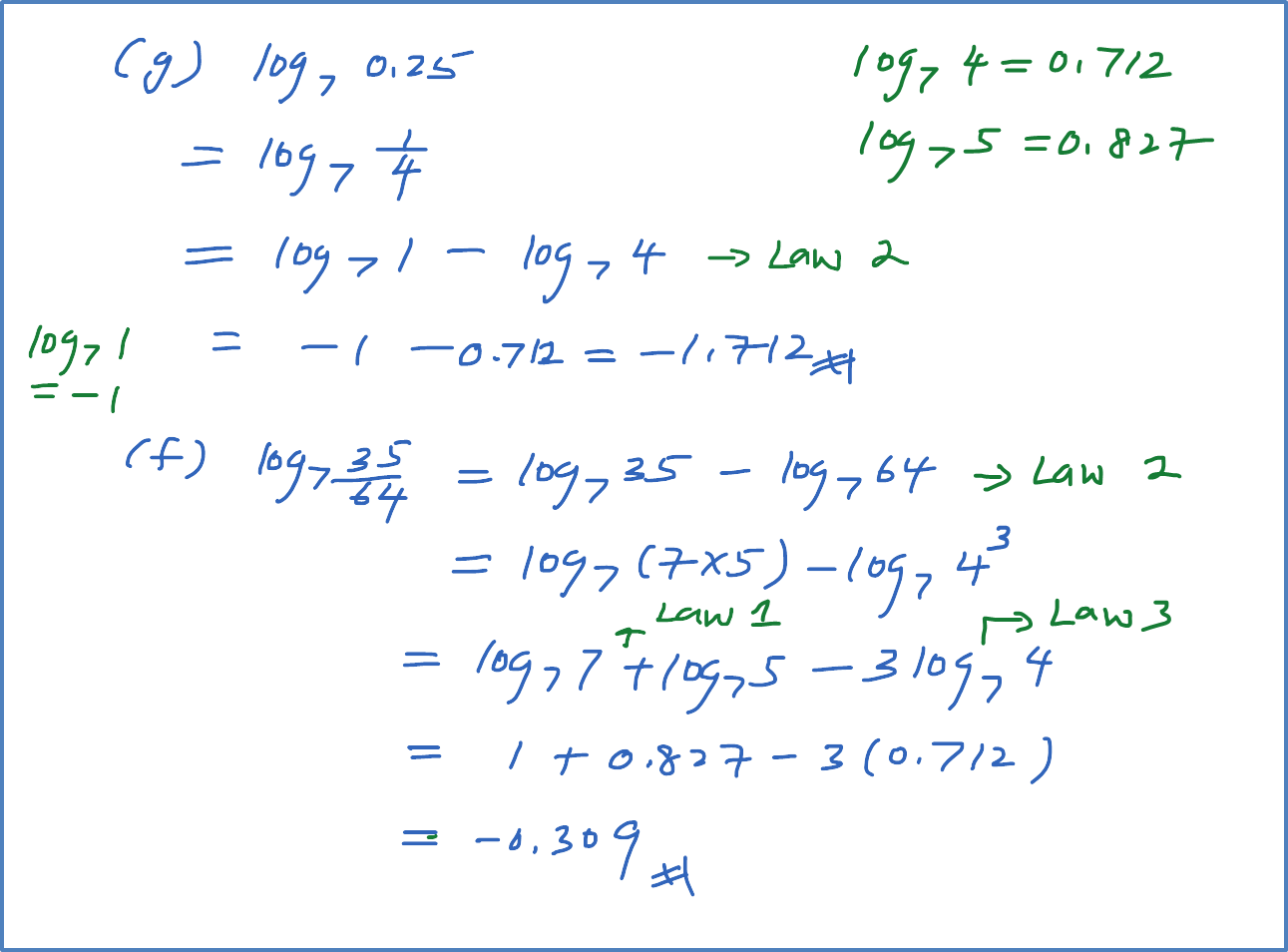\

# 4.2.1(c) Example 3 (Laws of Logarithms)

Example 3

Given that ${\mathrm{log}}_{7}4=0.712$   and ${\mathrm{log}}_{7}5=0.827$  , evaluate the following.
(a) ${\mathrm{log}}_{7}20$
(b) ${\mathrm{log}}_{7}1\frac{1}{4}$
(c) ${\mathrm{log}}_{7}0.8$
(d) ${\mathrm{log}}_{7}28$
(e) ${\mathrm{log}}_{7}140$
(f) ${\mathrm{log}}_{7}100$
(g) ${\mathrm{log}}_{7}0.25$
(h) ${\mathrm{log}}_{7}\frac{35}{64}$